# Simple Harmonic Motion Vidbook Pro SIMPLE HARMONIC MOTION Name Mech HW-79 Case A eum 1. Consider...

Simple Harmonic Motion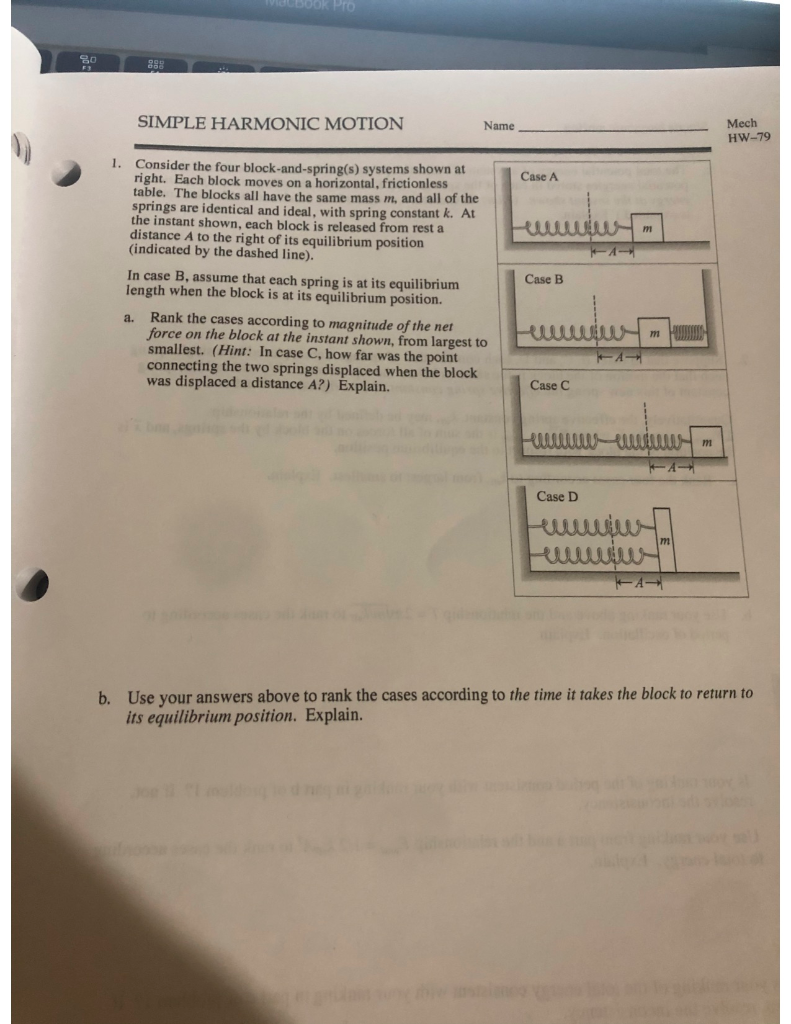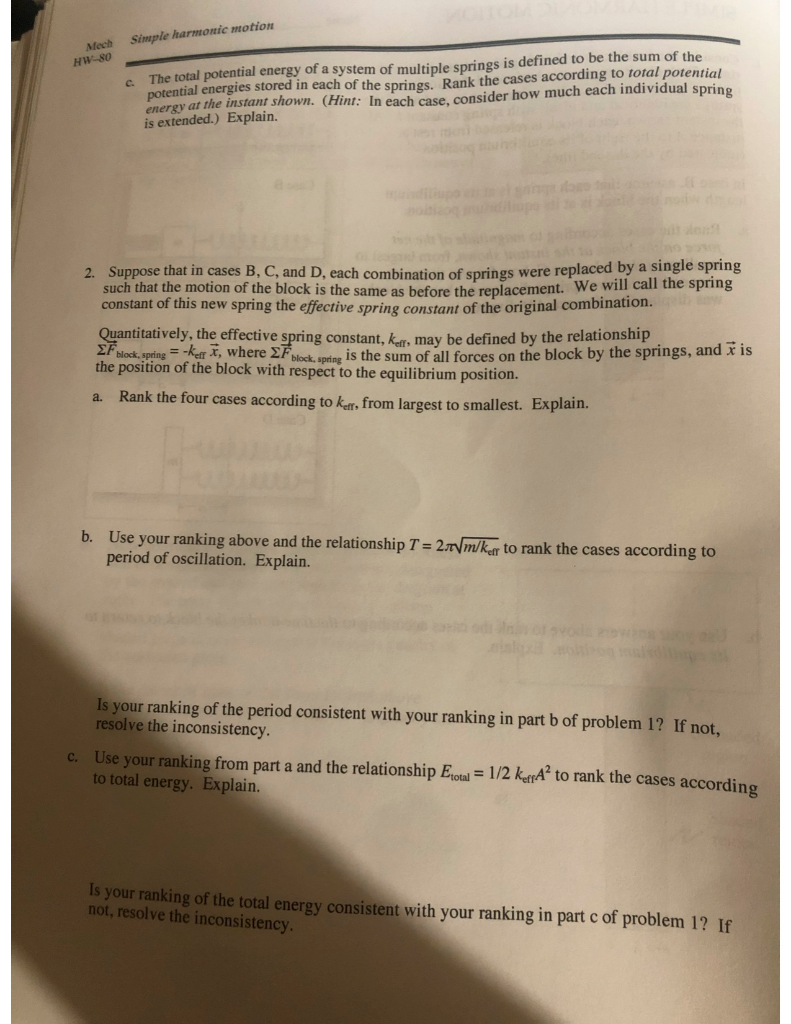Vidbook Pro SIMPLE HARMONIC MOTION Name Mech HW-79 Case A eum 1. Consider the four block-and-spring(s) systems shown at right. Each block moves on a horizontal, frictionless table. The blocks all have the same mass m, and all of the springs are identical and ideal, with spring constant k. At the instant shown, each block is released from rest a distance A to the right of its equilibrium position (indicated by the dashed line). In case B, assume that each spring is at its equilibrium length when the block is at its equilibrium position. a. Rank the cases according to magnitude of the net force on the block at the instant shown, from largest to smallest. (Hint: In case C, how far was the point connecting the two springs displaced when the block was displaced a distance A?) Explain. Case B Ulm Case C Hur U KA Case D Fute UUS b. Use your answers above to rank the cases according to the time it takes the block to return to its equilibrium position. Explain.
Mech Simple harmonic motion HW-80 The total potential energy of a system of multiple springs is defined to be the sum of the potential energies stored in each of the springs. Rank the cases according to total potential energy at the instant shown. (Hint: In each case, consider how much each individual spring is extended.) Explain. 2. Suppose that in cases B, C, and D. each combination of springs were replaced by a single spring such that the motion of the block is the same as before the replacement. constant of this new spring the effective spring constant of the original com Quantitatively, the effective spring constant, kar, may be defined by the relationship 2F block, spring = -keff X, where F block.spring is the sum of all forces on the block by the springs, and is the position of the block with respect to the equilibrium position. a. Rank the four cases according to kr, from largest to smallest. Explain. b. Use your ranking above and the relationship T = 2.7 m/ker to rank the cases according to period of oscillation. Explain. Is your ranking of the period consistent with your ranking in part b of problem 1? If not. resolve the inconsistency. c. Use your ranking from part a and the relationship Etotal = 1/2 keppA to rank the cases according to total energy. Explain. your ranking of the total energy consistent with your ranking in part c of problem 1? If not, resolve the inconsistency.

Ans 1)

a) Magnitude of force in a spring = kx, where k is spring constant and x is displacement

for case A

net force = kA

for case B

net force = kA + kA = 2kA

keff = 2k

for case C

the two springs are in series so keff = (1/k + 1/k)-1 = k/2

therefore net force = kA/2

for case D

the two springs are parallel so keff = k +k = 2k

Net force = 2kA

Rank D=B>A>C

b)

more force that means more acceleration which means lesser time to travel.

Which means force and time are inversely related

Rank C>A>D=B

c)

Work done by each springs in each case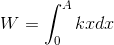for case A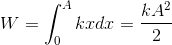for case B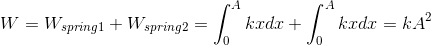for case C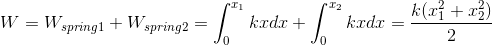x1+x2 =A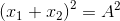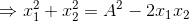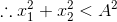this means potential energy of the two springs in case C is less than potential energy of the spring in Case A

for case DRank D=B>A>C

Ans 2

a)

effective spring constant is already found above for each case in Ans 1 a)

So, rank B=D>A>C

b)

Time period is inversely proportional to spring constant. So, more k means less T

So, rank C>A>D=B

c)

Ranking in terms of potential energy

we see the work done by each springs in the system

so potential energy = work done =1/2 kx2

For case A : potential energy of the spring = 1/2 kA2

For case B : potential energy = 1/2 *2k* A2 =-kA2

For case C : potential energy = 1/2 *k/2 * A2 = -1/4 kA2

For case D : potential energy = 1/2 * 2k* A2 =-kA2

Rank B=D>C>A

if any doubt feel free to comment

#### Earn Coin

Coins can be redeemed for fabulous gifts.

Similar Homework Help Questions
• ### Simple Harmonic Motion

When a 0.20-kg block is suspended from a vertically hanging spring, it stretches the spring from its unstrained 0.005 m. The same block is attached to the same springand placed on a horizontal, frictionless surface as shown. The block is then pulled so that the spring stretches a 0.11 m from its equilibrium position. The block isreleased and undergoes simple harmonic motion. What is the total mechanical energy of the system at any instant?

• ### A 55 kg block oscillates in simple harmonic motion on the end of a spring with...

A 55 kg block oscillates in simple harmonic motion on the end of a spring with a spring constant of 1500N/m according to xmcos (ωt+ φ). What is the shortest time it takes the block to move from position +0.800 xm to a position: +0.600 xm?

• ### Simple Harmonic MotionA block of mass 0.3 kg is attached to a spring of spring constant 28 N/m on a frictionless track. The block moves in simple harmonic motion with amplitude 0.12 m.While passing through the equilibrium point from left to right, the block is struck by a bullet, which stops inside the block. The velocity of the bulletimmediately before it strikes the block is 63 m/s and the mass of the bullet is 1.71 g.The collision occurred at the equilibrium position....

• ### Two separate masses on two separate springs undergo simple harmonic motion indefinitely (the surface is frictionless)....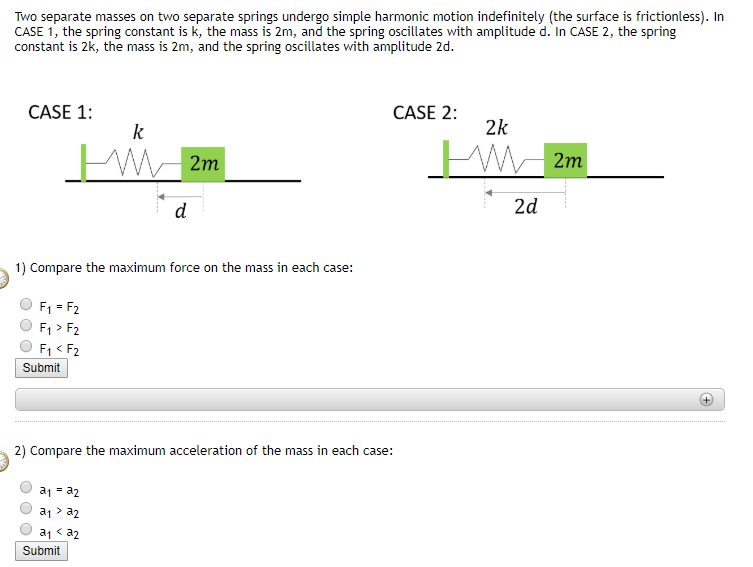Two separate masses on two separate springs undergo simple harmonic motion indefinitely (the surface is frictionless). In CASE 1, the spring constant is k, the mass is 2m, and the spring oscillates with amplitude d. In CASE 2, the spring constant is 2k, the mass is 2m, and the spring oscillates with amplitude 2d.  1) Compare the maximum force on the mass in each case: 2) Compare the maximum acceleration of the mass in each case: 3) Compare the total mechanical energy (potential...

• ### NEED HELP with...Energy of Harmonic OscillatorsLearning Goal: To learn to apply the law ofconservation of energy to the analysis of harmonic oscillators.Systems in simple harmonic motion, or harmonicoscillators, obey the law of conservation of energy just likeall other systems do. Using energyconsiderations, one can analyzemany aspects of motion of the oscillator. Such an analysis can besimplified if one assumes that mechanical energy is notdissipated.In other words,,where is the total mechanical energy of the system, is the kinetic energy, and is the potential energy.As you know,...

• ### 1) A 12.3 kg particle is undergoing simple harmonic motion with an amplitude of 1.86 mm....

1) A 12.3 kg particle is undergoing simple harmonic motion with an amplitude of 1.86 mm. The maximum acceleration experienced by the particle is 7.93 km/s2. (a) Find the period of the motion. (b) What is the maximum speed of the particle? (c) Calculate the total mechanical energy of this simple harmonic oscillator. 2) The orbit of the Moon around the Earth as projected along a diameter can be viewed as simple harmonic motion. Calculate the effective force constant k...

• ### Simple Harmonic Motion Consider a harmonic oscillator with period 0.13 s. If the speed at the...

Simple Harmonic Motion Consider a harmonic oscillator with period 0.13 s. If the speed at the equlibrium position is 2.10 m/s, what is the speed of a mass at a point which is displaced by 1.5 cm? (Work this out using conservation of energy). Please make sure to draw an energy bar chart for this. Find the answer and show the steps taken to get to the answer. Evaluate the answer and explain why it makes sense.

• ### Simple Harmonic Motion. Effective Spring Constant: In Part I you measured the keffective of the two...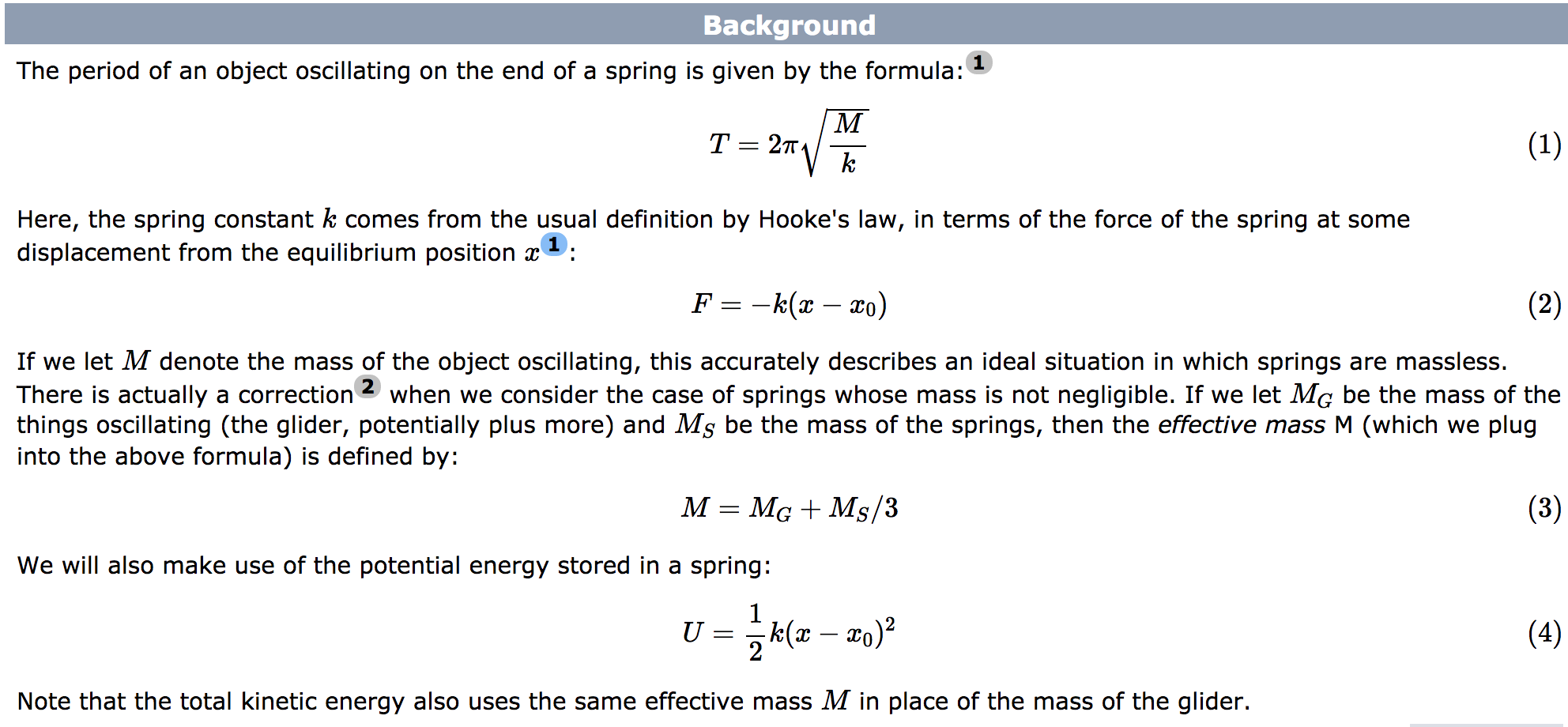Simple Harmonic Motion. Effective Spring Constant: In Part I you measured the keffective of the two springs acting together. If the two springs had k1 and k2 individually, how would they combine to get keff? Systemic Error: Leveling Air Track: We level the air track in this lab because it's good lab procedure in general, but in fact in this lab a not-level (but still straight) air track shouldn't change any of our results. Explain why this is true (for...

• ### RELATIVE MOTION Name Mech HW-27 The relationship Explain how this relation is consistent with your result...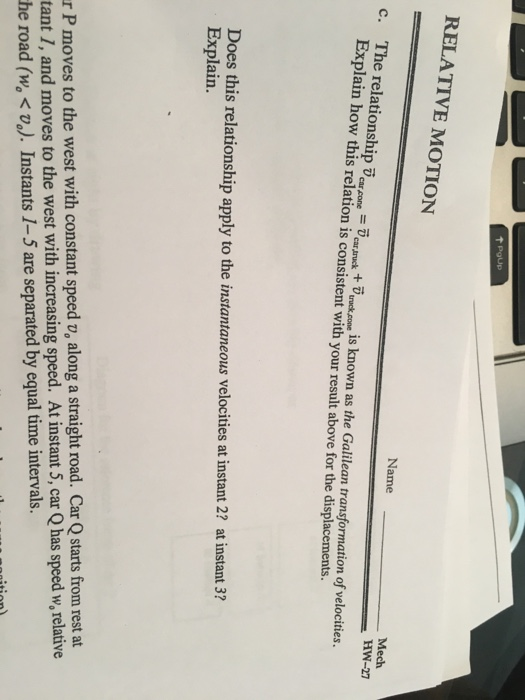RELATIVE MOTION Name Mech HW-27 The relationship Explain how this relation is consistent with your result above for the displacements. c. onea ruck t nck pow is known as the Galilean transformation of velocities. =- Does this relationship apply to the instantaneous velocities at instant 2? at instant 37 Explain. r P moves to the west with constant speed v, along a straight road. Car Q starts from rest at tant 1, and moves to the west with increasing speed....

• ### Homework for Lab 15: Simple Harmonic Motion Name Date Section 15 10 -5 -15-10-5 0 5...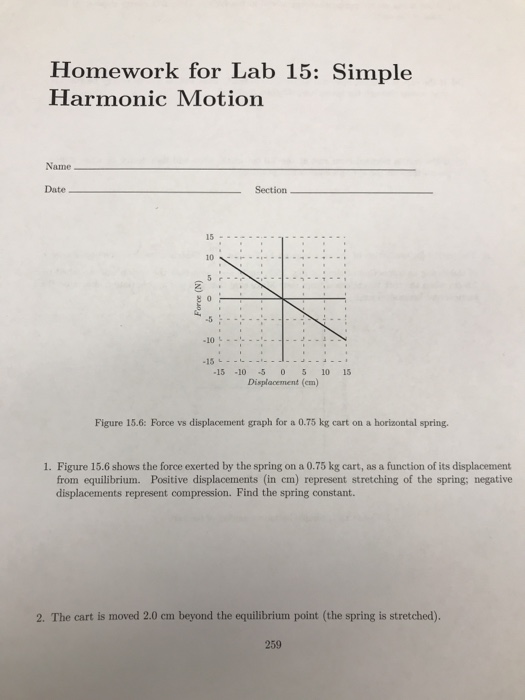Homework for Lab 15: Simple Harmonic Motion Name Date Section 15 10 -5 -15-10-5 0 5 10 15 Displacement (em) Figure 15.6: Force vs displacement graph for a 0.75 kg cart on a horizontal spring. 1. Figure 15.6 shows the force exerted by the spring on a 0.75 kg cart, as a function of its displacement from equilibrium. Positive displacements (in cm) represent stretching of the spring; negative displacements represent compression. Find the spring constant. 2. The cart is moved...